I took these notes while working through the Introduction to Time Series Analysis at DataCamp.

# Exploratory Analysis

The most useful way to view raw time series (`ts`) data is the `print()` function. It shows the observations and the Start, End, and Frequency. Function `length()` returns the number of observations. Functions `head()` and `tail()` print the first and last `n` observations. `str()` shows the structure.

Here are these functions used with the `Nile` dataset from the `datasets` package. `Nile` contains n = 100 measurements of the annual flow of the river Nile at Aswan, 1871–1970, in 10^8 m^3.

``print(Nile)``
``````## Time Series:
## Start = 1871
## End = 1970
## Frequency = 1
##    1120 1160  963 1210 1160 1160  813 1230 1370 1140  995  935 1110  994
##   1020  960 1180  799  958 1140 1100 1210 1150 1250 1260 1220 1030 1100
##    774  840  874  694  940  833  701  916  692 1020 1050  969  831  726
##    456  824  702 1120 1100  832  764  821  768  845  864  862  698  845
##    744  796 1040  759  781  865  845  944  984  897  822 1010  771  676
##    649  846  812  742  801 1040  860  874  848  890  744  749  838 1050
##    918  986  797  923  975  815 1020  906  901 1170  912  746  919  718
##    714  740``````
``length(Nile)``
``##  100``
``head(Nile, n = 10)``
``##   1120 1160  963 1210 1160 1160  813 1230 1370 1140``
``tail(Nile, n = 10)``
``##   1020  906  901 1170  912  746  919  718  714  740``

The `plot()` function is another useful way to explore the data. When there are multiple time series in the data, use `plot.ts()`. The default `xlab` is “Time”, so you will want to override it.

``````plot(Nile,
xlab = "Year",
ylab = "River Volume (1e9 m^{3})",
main = "Annual River Nile Volume at Aswan, 1871-1970",
type ="b")  # for points``````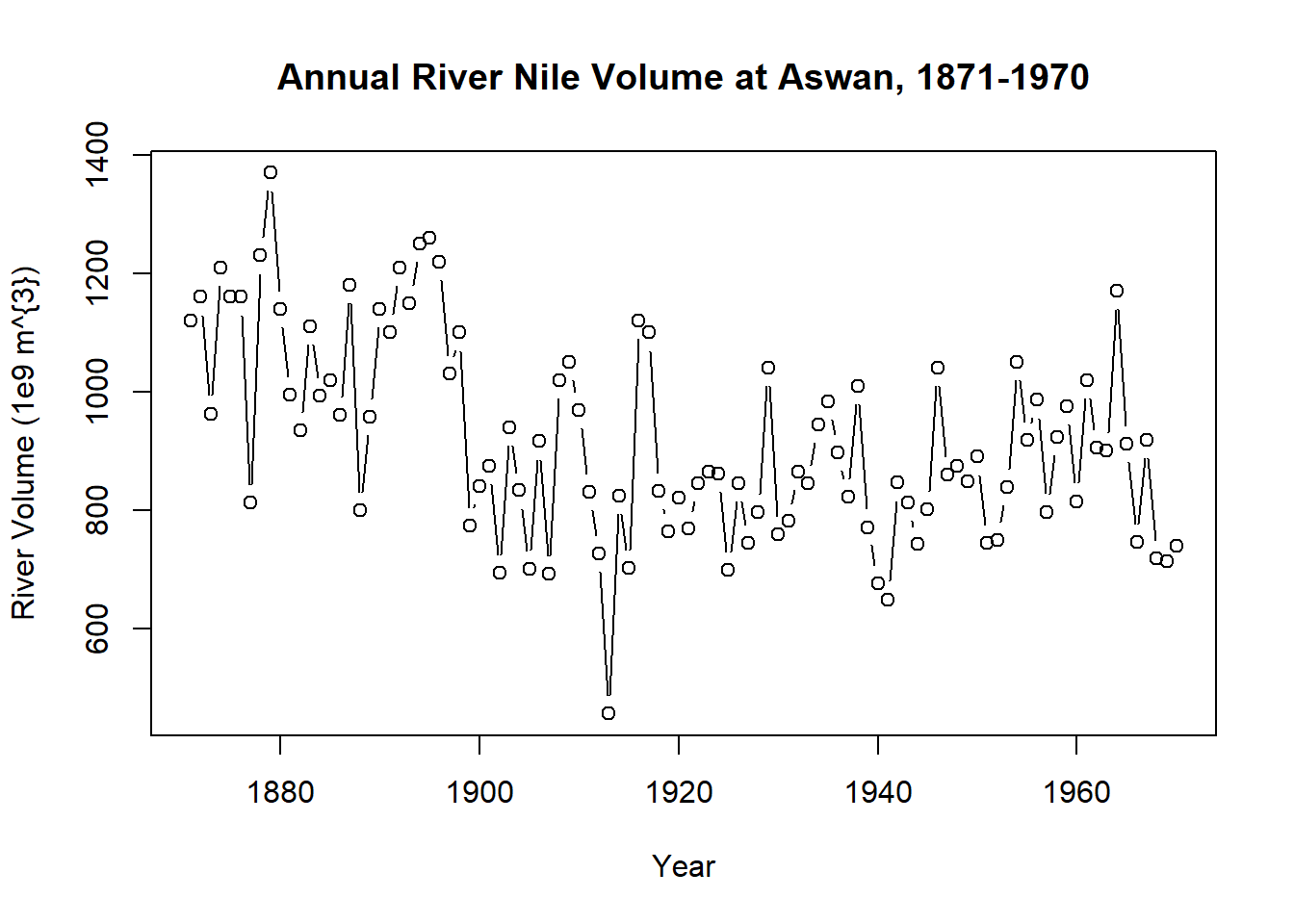The `deltat()` and `frequency()` functions show the spacing within a repeating series and number of periods within a series.

Here are these functions used with the `AirPassengers` dataset from the `datasets` package. `AirPassengers` contains n = 144 monthly totals of international airline passengers, 1949 to 1960.

``````plot(AirPassengers,
xlab = "Month",
ylab = "Passengers",
main = "International Airline Passengers, 1949-1960")``````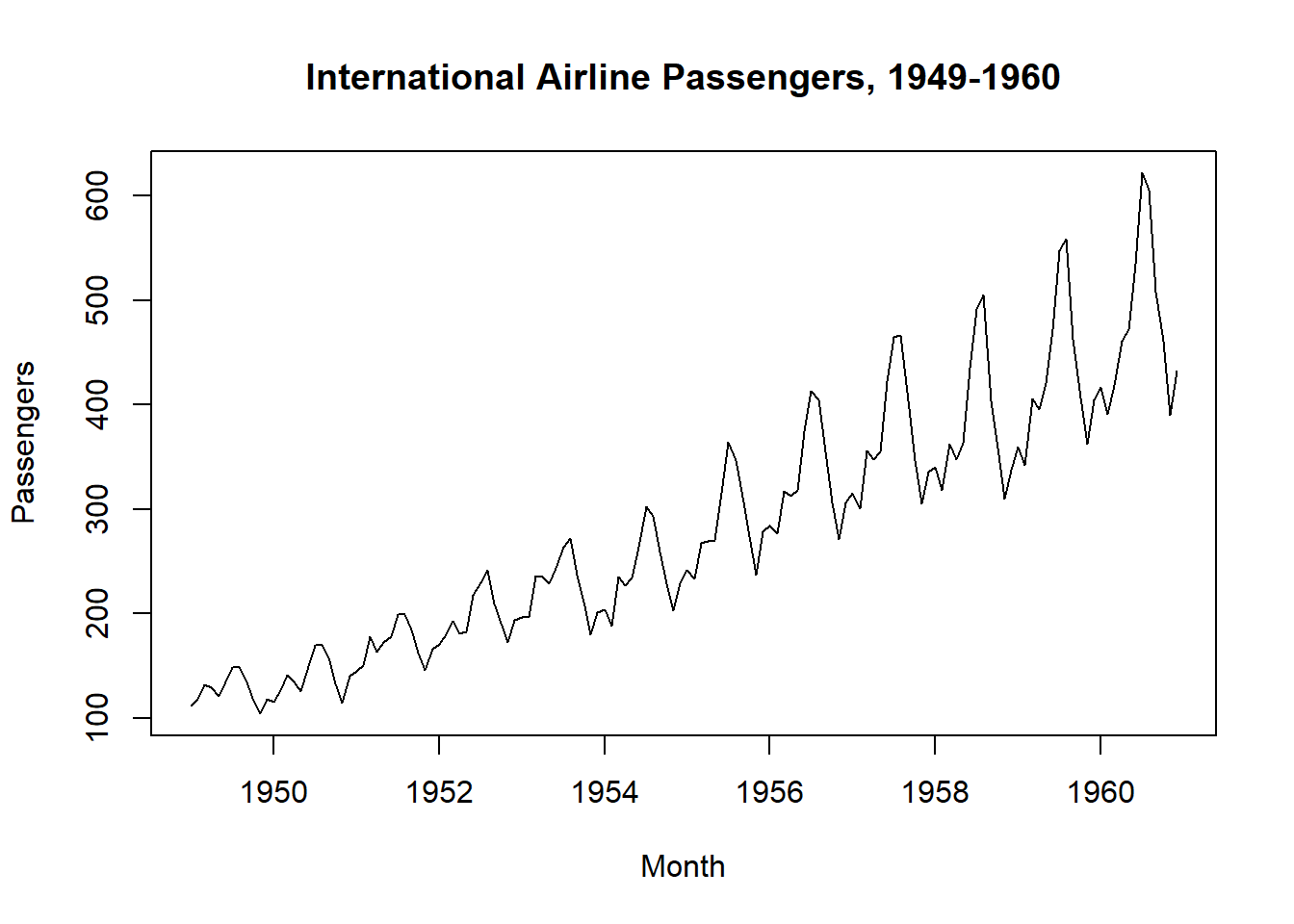``````# for monthly ts, points within year are separated by 1/12.
deltat(AirPassengers)``````
``##  0.08333333``
``````# Number of observations per period (12 months in year).
frequency(AirPassengers)``````
``##  12``
``````# retrieve the observation number within the cycle.
cycle(AirPassengers)``````
``````##      Jan Feb Mar Apr May Jun Jul Aug Sep Oct Nov Dec
## 1949   1   2   3   4   5   6   7   8   9  10  11  12
## 1950   1   2   3   4   5   6   7   8   9  10  11  12
## 1951   1   2   3   4   5   6   7   8   9  10  11  12
## 1952   1   2   3   4   5   6   7   8   9  10  11  12
## 1953   1   2   3   4   5   6   7   8   9  10  11  12
## 1954   1   2   3   4   5   6   7   8   9  10  11  12
## 1955   1   2   3   4   5   6   7   8   9  10  11  12
## 1956   1   2   3   4   5   6   7   8   9  10  11  12
## 1957   1   2   3   4   5   6   7   8   9  10  11  12
## 1958   1   2   3   4   5   6   7   8   9  10  11  12
## 1959   1   2   3   4   5   6   7   8   9  10  11  12
## 1960   1   2   3   4   5   6   7   8   9  10  11  12``````

If there are missing observations (`NA`s) in the time series, it is common practice to impute values with the time series mean.

Suppose `AirPassengers` is missing 12 months of data. The code below replaces the `NA`s with the mean and overlays the result in the original plot.

``````x <- AirPassengers
x[85:96] <- NA
plot(x)
x[which(is.na(x))] <- mean(x, na.rm = TRUE)
points(x, type = "l", col = 2, lty = 3)``````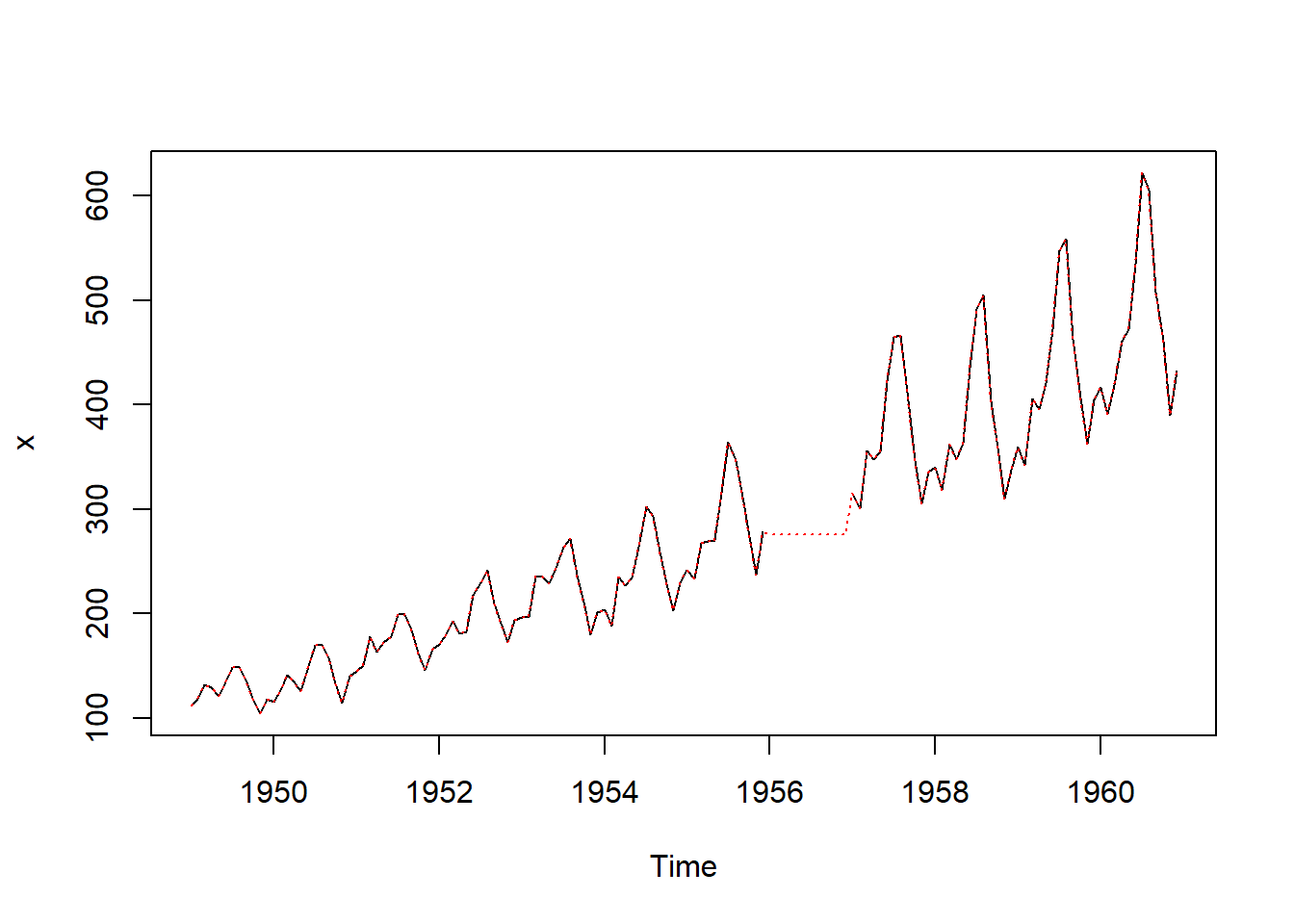The `ts()` function creates time series objects. A `ts` object is a vector or matrix with additional attributes, including time indices for each observation, the sampling frequency and time increment between observations, and the cycle length for periodic data.

If the time series is continuous, its points may or may not be evenly spaced. If it is discrete, the points are necesarily evenly spaced.

``````# Create a time series of quarterly data starting in 2017
x <- rnorm(n = 20)
x.ts <- ts(x,
start = 2017,
frequency = 4)
plot(x.ts,
xlab = "Quarter",
type = "b")``````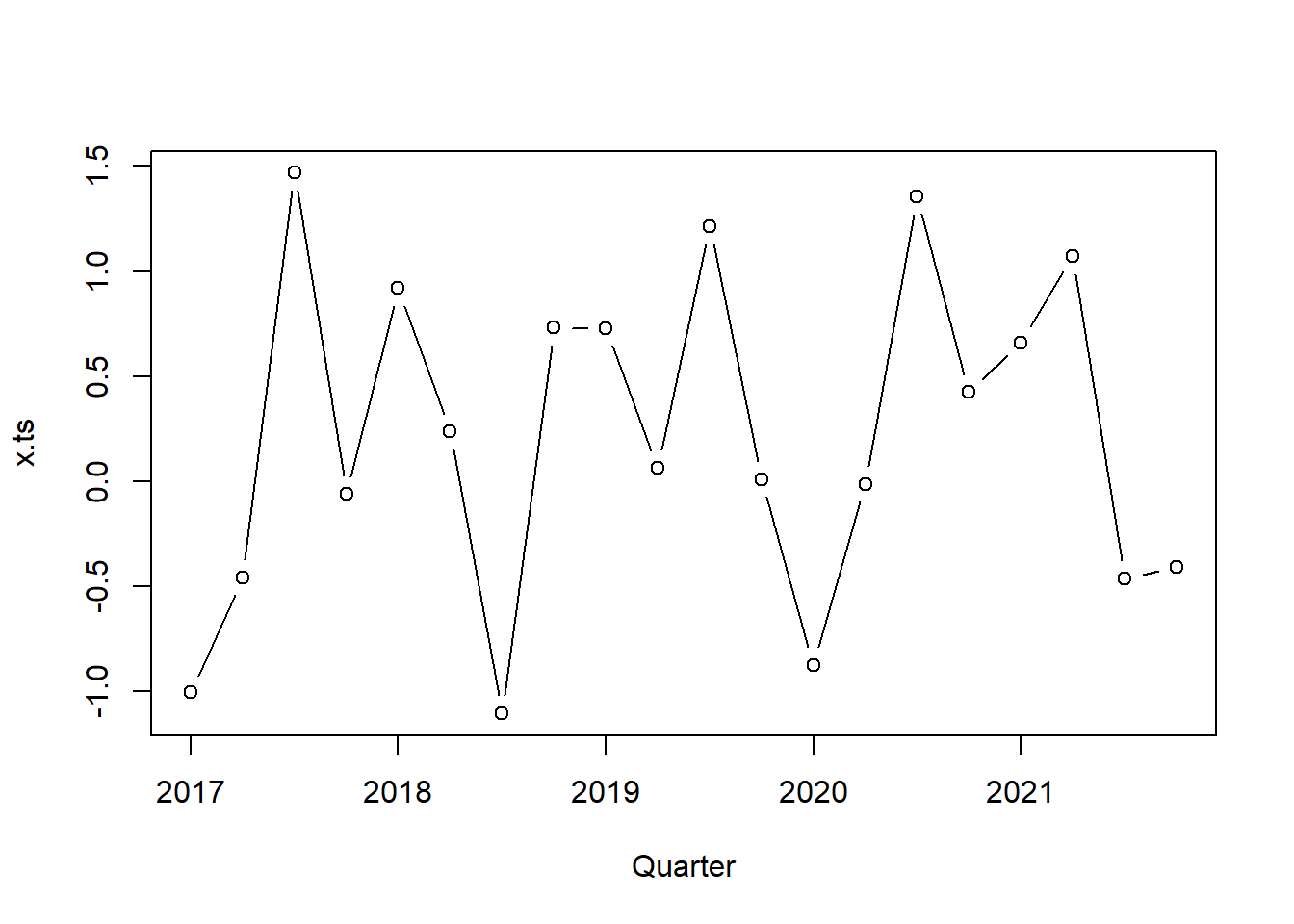The `xts` object is an alternative to `ts`. Create an `xts` object with `xts(x, order.by)` where `x` is the the data and `order.by` is a vector of dates/times to index the data.

``````library(xts)
x.xts <- xts(x,
order.by = seq(as.Date("2017-01-01"),
length = 20,
by = "quarters"))
plot(x.xts,
xlab = "Quarter",
type = "b")``````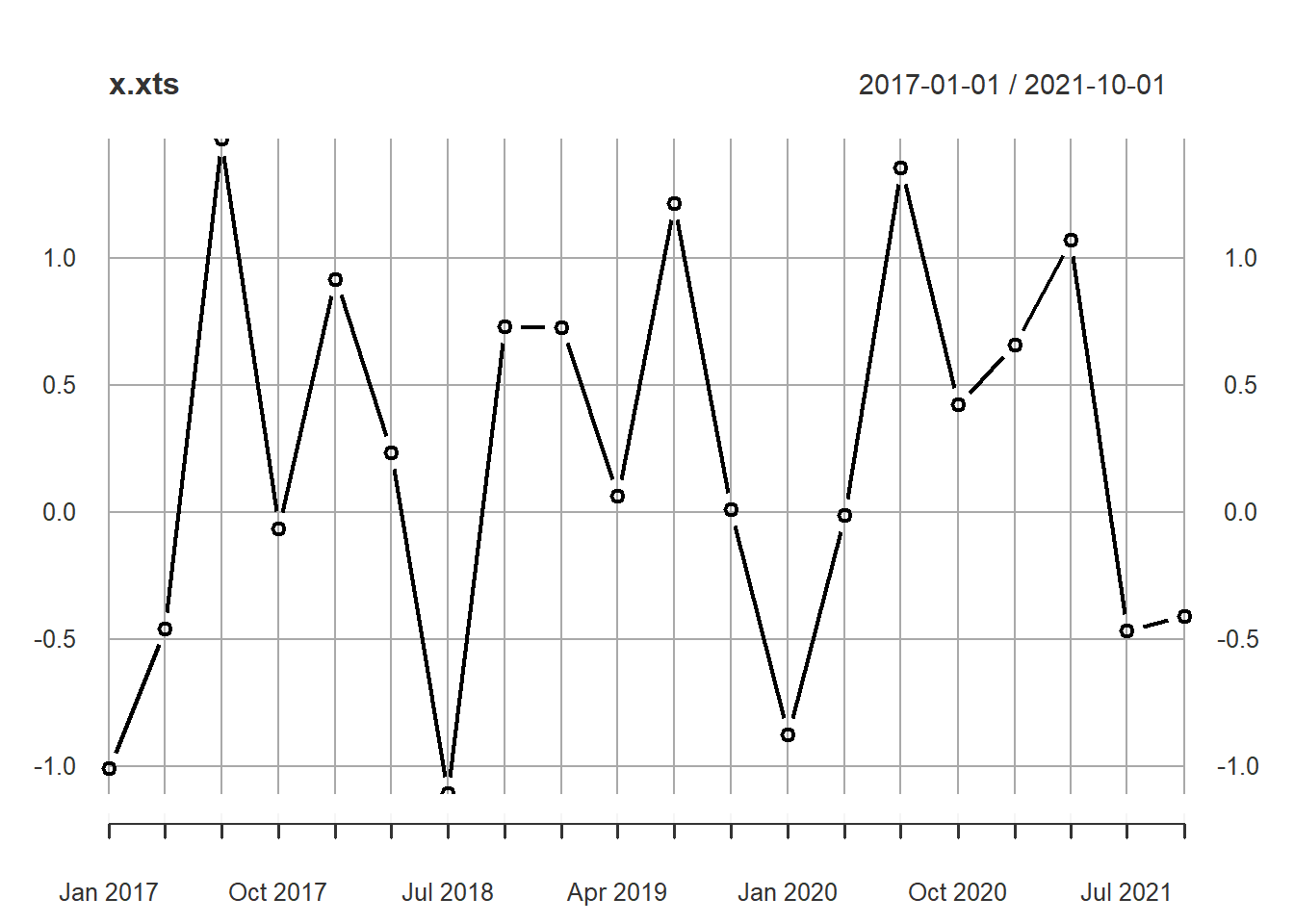If there are multiple time series in the data set, use the `ts.plot()` function instead of `plot()`. Dataset `EuStockMarkets` from the `datasets` package has multiple series. `EuStockMarkets` contains daily closing prices of major European stock indices from 1991-1998: Germany (`DAX`), Switzerland (`SMI`), France (`CAC`), and the UK (`FTSE`).

``````ts.plot(EuStockMarkets,
col = 1:4,
xlab = "Year",
ylab = "Index Value",
main = "Major European Stock Indices, 1991-1998")
legend("topleft",
colnames(EuStockMarkets),
lty = 1,
col = 1:4,
bty = "n")``````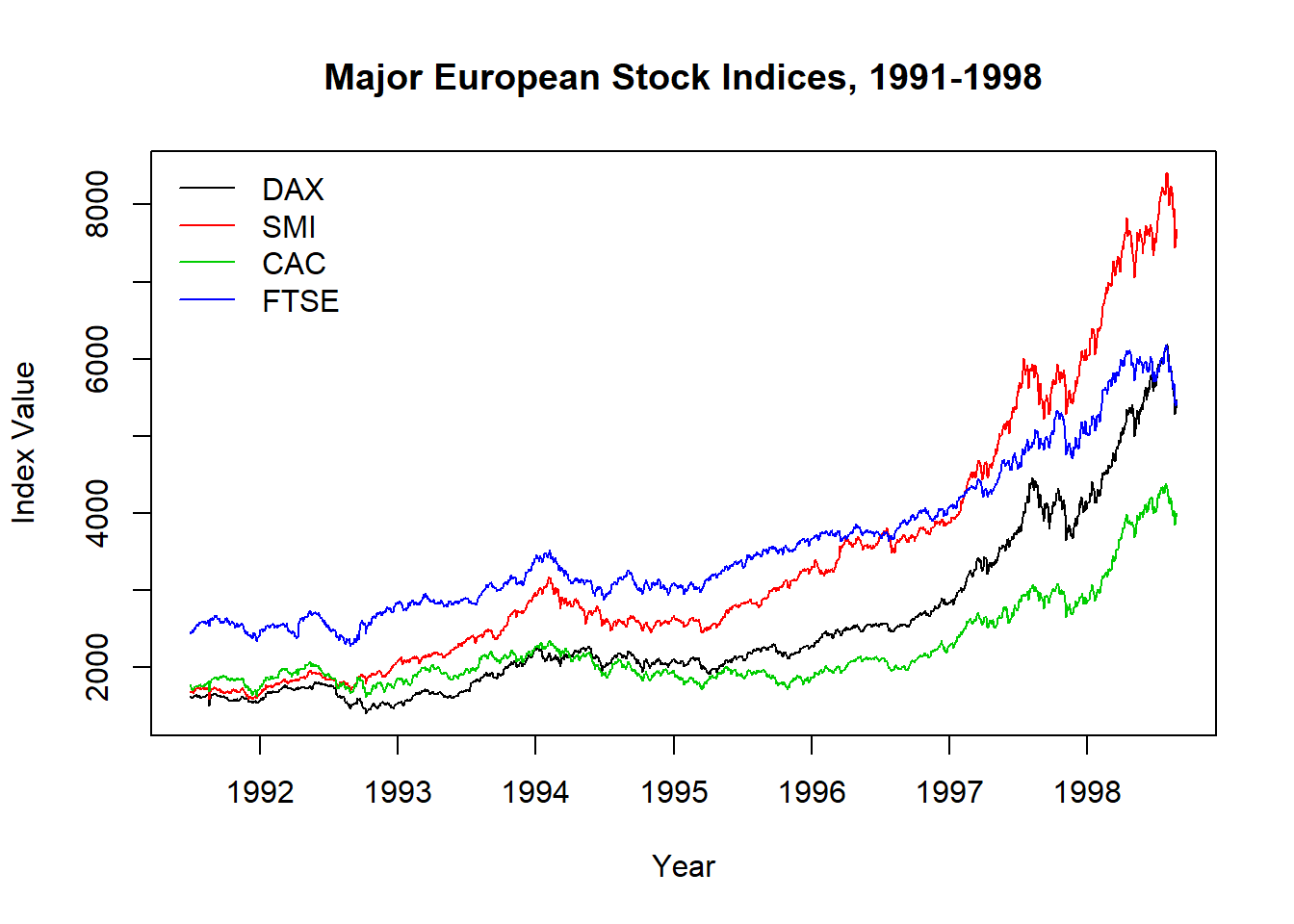# Prediction

The White Noise (WN) model is the simplest example of a stationary process. It has a fixed mean and variance. The WN model is one of several autoregressive integrated moving average (ARIMA) models. An ARIMA(p, d, q) model has three parts, the autoregressive order `p` (number of time lags), the order of integration (or differencing) `d`, and the moving average order `q`. When two out of the three terms are zeros, the model may be referred to based on the non-zero parameter, dropping “AR”, “I” or “MA” from the acronym describing the model. For example, ARIMA (1, 0,0) is AR(1), ARIMA(0,1,0) is I(1), and ARIMA(0,0,1) is MA(1). The WN model is ARIMA(0,0,0).

Simulate a WN time series using the `arima.sim()` function with argument `model = list(order = c(0, 0, 0))`. Here is a 50-period WN model with `mean` 100 and standard deviation `sd` of 10.

``````wn <- arima.sim(model = list(order = c(0, 0, 0)),
n = 50,
mean = 100,
sd = 10)
ts.plot(wn,
xlab = "Period",
ylab = "",
main = "WN Model, mean = 100, sd = 10")``````